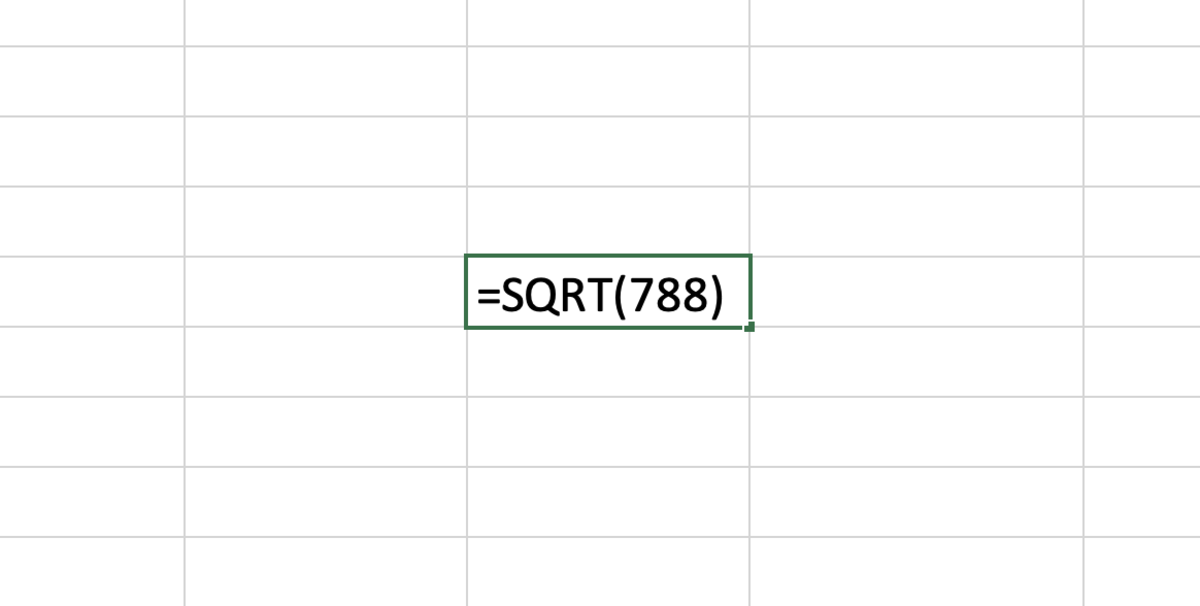# How to Use the SQRT Function in Excel

Joshua earned an MBA from USF and he writes mostly about software and technology.

## The Purpose of the SQRT Function

The SQRT function gives an Excel user the ability to find the positive decimal approximation of a square root. For example, this function can calculate the same problem shown in the illustration below.

## The SQRT Function Syntax

The SQRT function needs to be inputted into a cell like a formula for it work. To manually add this function the following steps need to be taken:

1. A cell needs clicked type "=SQRT(".
2. Lastly, add the number that you need to take the square root of following with a closed parenthesis.
3. After the formula is created the formula can be entered.

See below in bold the syntax of the SQRT function.

=SQRT(Number)

Number - A positive number. If the number used with this function is not positive an error will appear as the result.

## SQRT FunctionThe SQRT function gives an Excel user the ability to find the positive decimal approximation of a square root.

## SQRT Function Considerations

Using a Cell Reference

A cell reference with a value may my used instead of using a number in the formula.

=SQRT(A5)

Always Display a Positive Number

Since the number needs to be positive to avoid an error message using the ABS function helps you avoid this issue.

=ABS(SQRT(A5),"") or =ABS(SQRT(788),"")

Scroll to Continue

Using IFERROR

IF you would like to avoid an error message from a negative number or from a cell reference with no value you may elect to use the IFERROR function.

=IFFERROR((A5),"")

## Inserting the SQRT Function

The SQRT function can be inserted into a cell by selecting a cell followed by clicking the formula tab. Next, click on the math and trig functions button and choose the SQRT function from the list.

After the formula builder appears, add the number that you need the to find the square root for. A great feature of the formula builder is that you may change the number field to see different results.

To execute the results into the cell chosen click on done.

How to Use the COUNT Function in Excel

How to Use the AVERAGE Function in Excel

How to Use the ABS Function in Excel

How to Remove Errors in Excel With the IFERROR Function

How to Use the VLOOKUP Function in Excel

Converting Measurement Units: Using the CONVERT Function

Microsoft. (n.d.). SQRT function. Retrieved July 20, 2022, from https://support.microsoft.com/en-us/office/sqrt-function-654975c2-05c4-4831-9a24-2c65e4040fdf

To learn more about using functions in Excel I recommend The Excel 2019 Bible book. I have been using the Excel Bible for years to improve my understanding of all aspects of this Microsoft product.

This content is accurate and true to the best of the author’s knowledge and is not meant to substitute for formal and individualized advice from a qualified professional.